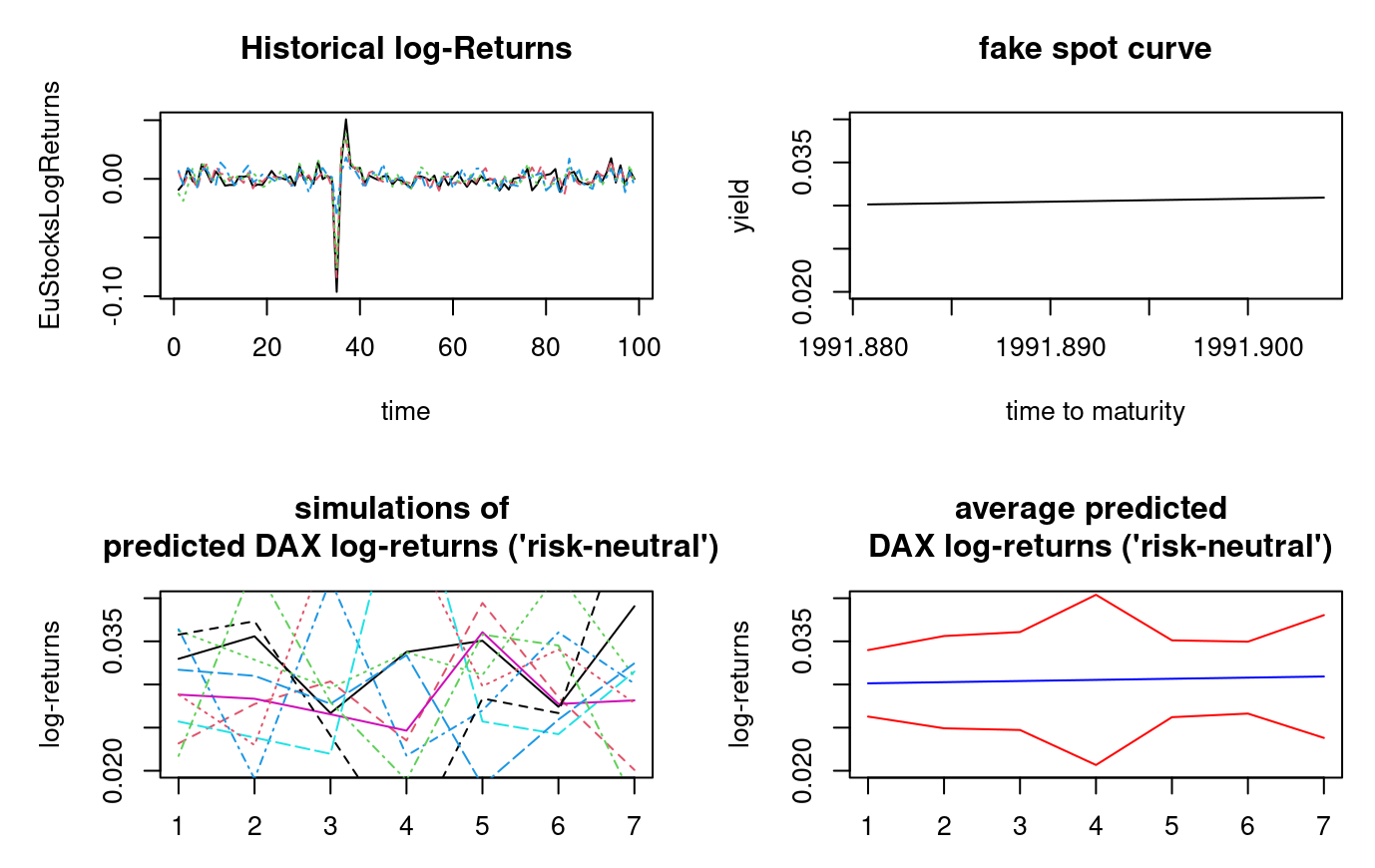Update 2023-09-07 and disclaimer : This is a work in progress, and some components are certainly missing. Submit an issue/discussion/pull request on GitHub if you want.

In R package ahead, it is possible to obtain simulations of risky assets returns both in historical and risk-neutral probability.

• 0 - Install ahead

• 1 - Get and transform data

• 2 - Risk-neutralize simulations

• 3 - Visualization

# 0 - Install ahead

ahead is released under the BSD Clear license. Here’s how to install the R version of the package:

• 1st method: from R-universe

In R console:

options(repos = c(
techtonique = 'https://techtonique.r-universe.dev',
CRAN = 'https://cloud.r-project.org'))


• 2nd method: from Github

In R console:

devtools::install_github("Techtonique/ahead")


Or

remotes::install_github("Techtonique/ahead")


Using ahead:

library(ahead)


# 1 - Get and transform data

data(EuStockMarkets)

EuStocks <- ts(EuStockMarkets[1:100, ],
start = start(EuStockMarkets),
frequency = frequency(EuStockMarkets))

EuStocksLogReturns <- ahead::getreturns(EuStocks, type = "log")



# 2 - Risk-neutralize simulations

## 2 - 1 Yield to maturities (fake risk-free rates)

ym <- c(0.03013425, 0.03026776, 0.03040053, 0.03053258, 0.03066390, 0.03079450, 0.03092437)

freq <- frequency(EuStocksLogReturns)
(start_preds <- tsp(EuStocksLogReturns) + 1 / freq)
(ym <- stats::ts(ym,
start = start_preds,
frequency = frequency(EuStocksLogReturns)))


## 2 - 2 Risk-neutralized simulations

obj <- ahead::ridge2f(EuStocksLogReturns, h = 7L,
type_pi = 'bootstrap',
B = 10L, ym = ym)


Checks

rowMeans(obj$neutralized_sims$CAC)

print(ym)

rowMeans(obj$neutralized_sims$DAX)

print(ym)


# 3 - Visualization


par(mfrow = c(2, 2))

matplot(EuStocksLogReturns, type = 'l',
main = "Historical log-Returns", xlab = "time")

plot(ym, main = "fake spot curve",
xlab = "time to maturity",
ylab = "yield",
ylim = c(0.02, 0.04))

matplot(obj$neutralized_sims$DAX, type = 'l',
main = "simulations of \n predicted DAX log-returns ('risk-neutral')",
ylim = c(0.02, 0.04),
ylab = "log-returns")

ci <- apply(obj$neutralized_sims$DAX, 1, function(x) t.test(x)$conf.int) plot(rowMeans(obj$neutralized_sims\$DAX), type = 'l', main = "average predicted \n DAX log-returns ('risk-neutral')", col = "blue",
ylim = c(0.02, 0.04),
ylab = "log-returns")
lines(ci[1, ], col = "red")
lines(ci[2, ], col = "red")